Next: About this document Up: My Home Page

# Sequential and Binary Search Lecture 19

Steven S. Skiena

Sequential Search

The simplest algorithm to search a dictionary for a given key is to test successively against each element.

This works correctly regardless of the order of the elements in the list. However, in the worst case all elements might have to be tested.

```Procedure Search(head:pointer, key:item):pointer;
Var
p:pointer;
found:boolean;
Begin
found:=false;
p:=head;
While (p # NIL) AND (not found) Do
Begin
If (p^.info = key) then
found = true;
Else
p = p^.next;
End;
return p;
END;```

With and Without Sentinels

A sentinel is a value placed at the end of an array to insure that the normal case of searching returns something even if the item is not found. It is a way to simplify coding by eliminating the special case.

```MODULE LinearSearch EXPORTS Main;  (*1.12.94. LB*)
(* Linear search without a sentinel *)

...

i:= FIRST(a);
WHILE (i <= last) AND NOT Text.Equal(a[i], x) DO INC(i) END;

IF i > last THEN
SIO.PutText("NOT found");
ELSE
SIO.PutText("Found at position: ");
SIO.PutInt(i)
END; (*IF i > last*)
SIO.Nl();
END LinearSearch.```

The sentinel insures that the search will eventually succeed:

```
MODULE SentinelSearch EXPORTS Main;      (*27.10.93. LB*)
(* Linear search with sentinel. *)

...

(* Do search *)
a[LAST(a)]:= x; (*sentinel at position N+1*)
i:= FIRST(a);
WHILE x # a[i] DO INC(i) END;

(* Output result *)
IF i = LAST(a) THEN
SIO.PutText("NOT found");
ELSE
SIO.PutText("Found at position: "); SIO.PutInt(i)
END;
SIO.Nl();
END SentinelSearch.```

Weighted Sequential Search

Sometimes sequential search is not a bad algorithm, especially when the list isn't long. After all, sequential search is easier to implement than binary search, and does not require the list to be sorted.

However, if we are going to do a sequential search, what order do we want the elements? Sorted order in a linked list doesn't really help, except maybe to help us stop early if the item isn't in the list.

Suppose you were organizing your personal phone book for sequential search. You would want your most frequently called friends to be at the front: In sequential search, you want the keys ordered by frequency of use!

Why? If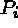is the probability of searching for the ith key, which is a distance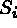from the front, the expected search time is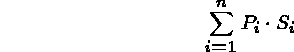which is minimized by placing the list in decreasing probability of access order.

For the list (Cheryl,0.4), (Lisa,0.25), (Lori,0.2), (Lauren,0.15), the expected search time is: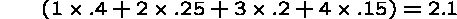If access probability had been uniform, the expected search time would have been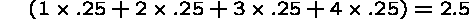So I win using this order, and win even more if the access probabilities are furthered skewed.

But how do I find the probabilities?

Self-Organizing Lists

Since it is often impractical to compute usage frequencies, and because usage frequencies often change in the middle of a program (locality), we would like our data structure to automatically adjust to the distribution.

Such data structures are called self-organizing.

The idea is to use a heuristic to move an element forward in the list whenever it is accessed. There are two possibilities:

• Move forward one is the ``conservative'' approach. (1,2,3,4,5) becomes (1,2,4,3,5) after a Find(4).
• Move to front is the ``liberal'' approach. (1,2,3,4,5) becomes (4,1,2,3,5) after a Find(4).

Which Heuristic is Better?

Move-forward-one can get caught in traps which won't fool move-to-front:

For list (1,2,3,4,5,6,7,8), the queries Find(8), Find(7), Find(8), Find(7), ... will search the entire list every time. With move-to-front, it averages only two comparisons per query!

In fact, it can be shown that the total search time with move-to-front is never more than twice the time if you knew the actual probabilities in advance!!

We will see self-organization again later in the semester when we talk about splay trees.

Let's Play 20 Questions!

```1.                       11.
2.                       12.
3.                       13.
4.                       14.
5.                       15.
6.                       16.
7.                       17.
8.                       18.
9.                       19.
10.                      20.```

Binary Search

Binary Search is an incredibly powerful technique for searching an ordered list. It is familiar to everyone who uses a telephone book!

The basic algorithm is to find the middle element of the list, compare it against the key, decide which half of the list must contain the key, and repeat with that half.

Two requirements to support binary search:

• Random access of the list elements, so we need arrays instead of linked lists.
• The array must contain elements in sorted order by the search key.

Why Do Twenty Questions Suffice?

With one question, I can distinguish between two words: A and B; ``Is the key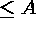?''

With two questions, I can distinguish between four words: A,B,C,D; ``Is the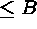?''

Each question I ask em doubles the number of words I can search in my dictionary.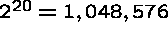, which is much larger than any portable dictionary!

Thus I could waste my first two questions because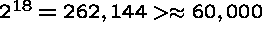.

Exponents and Logs

Recall the definitions of exponent and logarithm from high school: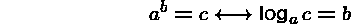Thus exponentiation and logarithms are inverse functions, since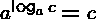.

Note that the logarithm of a big number is a much smaller number.

Thus the number of questions we must ask is the base two logarithm of the size of the dictionary.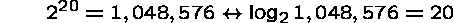Implementing Binary Search

Although the algorithm is simple to describe informally, it is tricky to produce a working binary search function. The first published binary search algorithm appeared in 1946, but the first correct published program appeared in 1962!

The difficulty is maintaining the following two invariants with each iteration:

• The key must always remain between the low and high indices.
• The low or high indice must advance each iteration.

The boundary cases are very tricky: zero elements left, one elements left, two elements left, and an even or odd number of elements!

Versions of Binary Search

There are at least two different versions of binary search, depending upon whether we want to test for equality at each query or only at the end.

For the later, suppose we want to search for ``k'':

```iteration  bottom top      mid
---------------------------------------
1          2      14       (1+14)/2=7
2          1      7        (1+7)/2=4
3          5      7        (5+7)/2=6
4          6      7        (7+7)/2=7```

Since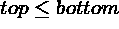, 7 is the right spot. However, we must now test if entry='k'. If not, the item isn't in the array.

Alternately, we can test for equality at each comparison. Suppose we search for ``c'':

```iteration  bottom  top  mid
------------------------------------
1          1       14   (1+14)/2 = 7
2          1       6    (1+6)/2 = 3
3          1       2    (1+2)/2 = 1
4          2       2    (2+2)/2 = 2```

Now it will be found!

Recursive Binary Search Implementation

```PROCEDURE Search( READONLY array: ARRAY [0 .. MaxInd - 1] OF INTEGER;
left, right: [0 .. MaxInd - 1];
argument: INTEGER): [0..MaxInd] =
(*Implements binary search in an array*)
VAR
middle := left + (right - left) DIV 2;

BEGIN (* binary search *)
IF argument = array[middle] THEN      (*found*)
RETURN middle
ELSIF argument < array[middle] THEN   (*search in left half*)
IF left < middle THEN
RETURN Search(array, left, middle - 1, argument)
ELSE                                (*left boundary reaches middle: not found*)
RETURN MaxInd
END (*IF left < middle*)
ELSE                                  (*search in right half*)
IF middle < right THEN
RETURN Search(array, middle + 1, right, argument)
ELSE                                (*middle reaches right boundary: not found*)
RETURN MaxInd
END (*IF middle < right*)
END (*IF argument = array[middle]*)
END Search;```Next: About this document Up: My Home Page

Steve Skiena
Thu Oct 30 10:36:28 EST 1997